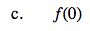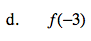### Home > CA > Chapter Ch12 > Lesson 12.3.1 > Problem12-57

12-57.
1. Use the graph of f(x) below to find the following values. Homework Help ✎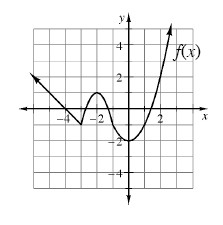1. f(1)

2. f(−6)

3. f(0)

4. f(−3)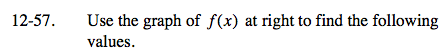Find the point on the function that has an x-value equal to the input. Its y-value will equal the output.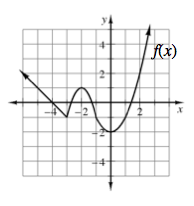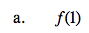f(1) = −1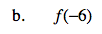f(−6) = 2